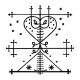pmr -4 years ago 115
C++ Question

# Iterators for circular structures

The following code shows what I currently have. It is an adapter for
circular data-structures. The main function shows how it is used. This
is all nice and fast but I really would like to have iterators over
the structure defined by

`circ`
. All approaches up till now involved
either some counting scheme (count the range if with the circulator,
build an iterator that counts increments and decrements) or boolean
values to check if the iterator has been moved to avoid begin and end
being equal.

Are there some common solutions to adapt a circular structure to
iterators? What other work-arounds are possible?

I would like to preserve the iteration speed as much as possible but
am willing to make compromises here. I would value a fully conforming
iterator over a minor speed penalty.

``````#include <cstddef> // nullptr
#include <iostream>
#include <boost/noncopyable.hpp>
#include <boost/operators.hpp>

// circular structure that we want to iterate over
struct circ : private boost::noncopyable {
unsigned int i;
circ* next;
circ* prev;
};

// whacked up circulator, imagine some template cream here to make it
// generic, omitted to preserve sanity
struct circulator
: public boost::incrementable<circulator>
, public boost::decrementable<circulator>
, public boost::equality_comparable<circulator, circulator>
, public boost::dereferenceable<circulator, circ*>
{
circulator()
: c(nullptr) {}
circulator(circ& c) : c(&c) {}

bool operator==(const circulator& other) const {
return this->c == other.c;
}

circulator& operator++() { c = c->next; return *this; }
circulator& operator--() { c = c->prev; return *this; }

explicit operator bool() const { return c; }

circ& operator*() const { return *c; }

circ* c;
};

int main()
{
circ a, b, c, d;
a.next = &b; a.prev = &a; a.i = 0;
b.next = &c; b.prev = &a; b.i = 1;
c.next = &d; c.prev = &b; c.i = 2;
d.next = &a; d.prev = &c; d.i = 3;
circulator begin{a}, end{a};
if(begin)
do {
std::cout << begin->i << std::endl;
begin = ++begin;
} while(begin != end);

return 0;
}
``````

If need be I can add some of my previous approaches, but they are
rather verbose and would add unnecessary bloat to the question.

EDIT: It would be nice if the resulting iterator would be bidirectional. Although I can give up this requirement.Robᵩ
Answer Source

If it were me, I'd have `operator++` notice the terminal condition, and set `c` to some sentinal value:

``````circulator(circ& c) : c(&c), start(&c) {}
circulator& operator++() { c = c->next; if(c==start) c=nullptr; return *this; }
``````

Usage:

``````circulator begin{a}, end;
while(begin != end) {
begin++;
}
``````

Note that this usage defines the end iterator as holding a nullptr, which means that you can't do this:

``````circulator end;
--end;
``````
Recommended from our users: Dynamic Network Monitoring from WhatsUp Gold from IPSwitch. Free Download
Latest added